# Speaker Recognition Using x-vectors

Speaker recognition answers the question "Who is speaking?". Speaker recognition is usually divided into two tasks: speaker identification and speaker verification. In speaker identification, a speaker is recognized by comparing their speech to a closed set of templates. In speaker verification, a speaker is recognized by comparing the likelihood that the speech belongs to a particular speaker against a predetermined threshold. Traditional machine learning methods perform well at these tasks in ideal conditions. For examples of speaker identification using traditional machine learning methods, see Speaker Identification Using Pitch and MFCC and Speaker Verification Using i-Vectors. Audio Toolbox™ provides `ivectorSystem` which encapsulates the ability to train an i-vector system, enroll speakers or other audio labels, evaluate the system for a decision threshold, and identify or verify speakers or other audio labels.

In adverse conditions, the deep learning approach of x-vectors has been shown to achieve state of the art results for many scenarios and applications . The x-vector system is an evolution of i-vectors originally developed for the task of speaker verification.

In this example, you develop an x-vector system. First, you train a time-delay neural network (TDNN) to perform speaker identification. Then you train the traditional backends for an x-vector-based speaker verification system: an LDA projection matrix and a PLDA model. You then perform speaker verification using the TDNN and the backend dimensionality reduction and scoring. The x-vector system backend, or classifier, is the same as developed for i-vector systems. For details on the backend, see Speaker Verification Using i-Vectors and `ivectorSystem`.

In Speaker Diarization Using x-vectors, you use the x-vector system trained in this example to perform speaker diarization. Speaker diarization answers the question "Who spoke when?".

Throughout this example, you will find live controls on tunable parameters. Changing the controls does not rerun the example. If you change a control, you must rerun the example.

### Data Set Management

This example uses the Pitch Tracking Database from Graz University of Technology (PTDB-TUG) . The data set consists of 20 English native speakers reading 2342 phonetically rich sentences from the TIMIT corpus. Download and extract the data set. Depending on your system, downloading and extracting the data set can take approximately 1.5 hours.

```url = 'https://www2.spsc.tugraz.at/databases/PTDB-TUG/SPEECH_DATA_ZIPPED.zip'; downloadFolder = tempdir; datasetFolder = fullfile(downloadFolder,'PTDB-TUG'); if ~exist(datasetFolder,'dir') disp('Downloading PTDB-TUG (3.9 G) ...') unzip(url,datasetFolder) end```

Create an `audioDatastore` object that points to the data set. The data set was originally intended for use in pitch-tracking training and evaluation, and includes laryngograph readings and baseline pitch decisions. Use only the original audio recordings.

```ads = audioDatastore([fullfile(datasetFolder,"SPEECH DATA","FEMALE","MIC"),fullfile(datasetFolder,"SPEECH DATA","MALE","MIC")], ... 'IncludeSubfolders',true, ... 'FileExtensions','.wav'); fileNames = ads.Files;```

Read an audio file from the training data set, listen to it, and then plot it.

```[audioIn,audioInfo] = read(ads); fs = audioInfo.SampleRate; t = (0:size(audioIn,1)-1)/fs; sound(audioIn,fs) plot(t,audioIn) xlabel('Time (s)') ylabel('Amplitude') axis([0 t(end) -1 1]) title('Sample Utterance from Training Set')```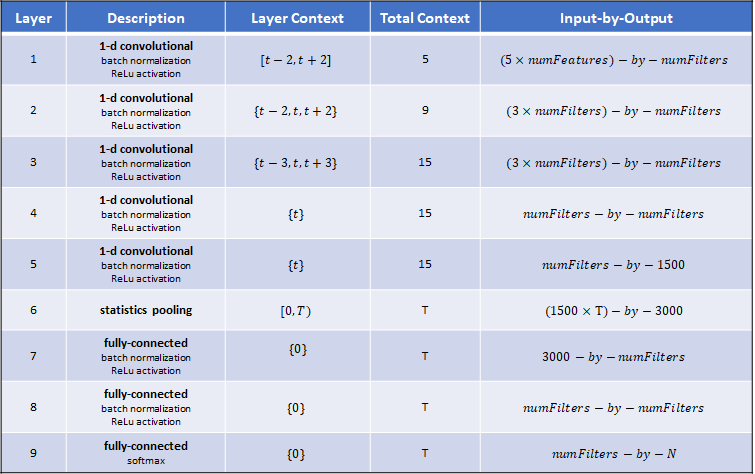The file names contain the speaker IDs. Decode the file names to set the labels on the `audioDatastore` object.

```speakerIDs = extractBetween(fileNames,'mic_','_'); ads.Labels = categorical(speakerIDs);```

Separate the `audioDatastore` object into five sets:

• `adsTrain` - Contains training set for the TDNN and backend classifier.

• `adsValidation` - Contains validation set to evaluate TDNN training progress.

• `adsTest` - Contains test set to evaluate the TDNN performance for speaker identification.

• `adsEnroll` - Contains enrollment set to evaluate the detection error tradeoff of the x-vector system for speaker verification.

• `adsDET` - Contains evaluation set used to determine the detection error tradeoff of the x-vector system for speaker verification.

```developmentLabels = categorical(["M01","M02","M03","M04","M06","M07","M08","M09","F01","F02","F03","F04","F06","F07","F08","F09"]); evaluationLabels = categorical(["M05","M010","F05","F010"]); adsTrain = subset(ads,ismember(ads.Labels,developmentLabels)); [adsTrain,adsValidation,adsTest] = splitEachLabel(adsTrain,0.8,0.1,0.1); adsEvaluate = subset(ads,ismember(ads.Labels,evaluationLabels)); [adsEnroll,adsDET] = splitEachLabel(adsEvaluate,3);```

Display the label distributions of the resulting `audioDatastore` objects.

`countEachLabel(adsTrain)`
```ans=16×2 table Label Count _____ _____ F01 170 F02 170 F03 170 F04 170 F06 170 F07 170 F08 168 F09 170 M01 170 M02 170 M03 170 M04 170 M06 170 M07 170 M08 170 M09 170 ```
`countEachLabel(adsValidation)`
```ans=16×2 table Label Count _____ _____ F01 22 F02 22 F03 22 F04 22 F06 22 F07 22 F08 21 F09 22 M01 22 M02 22 M03 22 M04 22 M06 22 M07 22 M08 22 M09 22 ```
`countEachLabel(adsTest)`
```ans=16×2 table Label Count _____ _____ F01 21 F02 21 F03 21 F04 21 F06 21 F07 21 F08 21 F09 21 M01 21 M02 21 M03 21 M04 21 M06 21 M07 21 M08 21 M09 21 ```
`countEachLabel(adsEnroll)`
```ans=2×2 table Label Count _____ _____ F05 3 M05 3 ```
`countEachLabel(adsDET)`
```ans=2×2 table Label Count _____ _____ F05 231 M05 231 ```

You can reduce the training and detection error trade-off datasets used in this example to speed up the runtime at the cost of performance. In general, reducing the data set is a good practice for development and debugging.

```speedUpExample =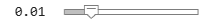false; if speedUpExample adsTrain = splitEachLabel(adsTrain,20); adsDET = splitEachLabel(adsDET,20); end```

### Feature Extraction

Create an `audioFeatureExtractor` object to extract 30 MFCCs from 30 ms Hann windows with a 10 ms hop. The sample rate of the data set is 48 kHz, but you will downsample the data set to 16 kHz. Design the `audioFeatureExtractor` assuming the desired sample rate, 16 kHz.

```desiredFs = 16e3; windowDuration =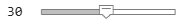0.03; hopDuration =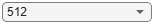0.005; windowSamples = round(windowDuration*desiredFs); hopSamples = round(hopDuration*desiredFs); overlapSamples = windowSamples - hopSamples; numCoeffs =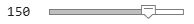30; afe = audioFeatureExtractor( ... 'SampleRate',desiredFs, ... 'Window',hann(windowSamples,'periodic'), ... 'OverlapLength',overlapSamples, ... ... 'mfcc',true, ... 'pitch',false, ... 'spectralEntropy',false, ... 'spectralFlux',false); setExtractorParams(afe,'mfcc','NumCoeffs',numCoeffs)```

Downsample the audio data to 16 kHz and extract features from the train and validation data sets. Use the training data set to determine the mean and standard deviation of the features to perform feature standardization. The supporting function, xVectorPreprocessBatch, uses your default parallel pool if you have Parallel Computing Toolbox™.

```adsTrain = transform(adsTrain,@(x)resample(x,desiredFs,fs)); [features,YTrain] = xVectorPreprocessBatch(adsTrain,afe); featuresMAT = cat(1,features{:}); numFeatures = size(featuresMAT,2); factors = struct('Mean',mean(featuresMAT,1),'STD',std(featuresMAT,1)); XTrain = cellfun(@(x)(x-factors.Mean)./factors.STD,features,'UniformOutput',false); XTrain = cellfun(@(x)x-mean(x(:)),XTrain,'UniformOutput',false); adsValidation = transform(adsValidation,@(x)resample(x,desiredFs,fs)); [XValidation,YValidation] = xVectorPreprocessBatch(adsTrain,afe,'Factors',factors); classes = unique(YTrain); numClasses = numel(classes);```

### x-vector Feature Extraction Model

In this example, you implement the x-vector feature extractor model  using the functional programming paradigm provided by Deep Learning Toolbox™. This paradigm enables complete control of the design of your deep learning model. For a tutorial on functional programming in Deep Learning Toolbox, see Define Model Gradients Function for Custom Training Loop (Deep Learning Toolbox). The supporting function, `xvecModel`, is placed in your current folder when you open this example. Display the contents of the `xvecModel` function.

`type('xvecModel')`
```function [Y,state] = xvecModel(X,parameters,state,nvargs) % This function is only for use in this example. It may be changed or % removed in a future release. arguments X parameters state nvargs.DoTraining = false nvargs.OutputLayer = 'final' nvargs.Dropout = 0.2; end % LAYER 1 ----------------------------------------------------------------- Y = dlconv(X,parameters.conv1.Weights,parameters.conv1.Bias,'DilationFactor',1); if nvargs.DoTraining [Y,state.batchnorm1.TrainedMean,state.batchnorm1.TrainedVariance] = ... batchnorm(Y, ... parameters.batchnorm1.Offset, ... parameters.batchnorm1.Scale, ... state.batchnorm1.TrainedMean, ... state.batchnorm1.TrainedVariance); Y(rand(size(Y))<nvargs.Dropout) = 0; else Y = batchnorm(Y, ... parameters.batchnorm1.Offset, ... parameters.batchnorm1.Scale, ... state.batchnorm1.TrainedMean, ... state.batchnorm1.TrainedVariance); end if nvargs.OutputLayer==1 return end Y = relu(Y); % ------------------------------------------------------------------------- % LAYER 2 ----------------------------------------------------------------- Y = dlconv(Y,parameters.conv2.Weights,parameters.conv2.Bias,'DilationFactor',2); if nvargs.DoTraining [Y,state.batchnorm2.TrainedMean,state.batchnorm2.TrainedVariance] = ... batchnorm(Y, ... parameters.batchnorm2.Offset, ... parameters.batchnorm2.Scale, ... state.batchnorm2.TrainedMean, ... state.batchnorm2.TrainedVariance); Y(rand(size(Y))<nvargs.Dropout) = 0; else Y = batchnorm(Y, ... parameters.batchnorm2.Offset, ... parameters.batchnorm2.Scale, ... state.batchnorm2.TrainedMean, ... state.batchnorm2.TrainedVariance); end if nvargs.OutputLayer==2 return end Y = relu(Y); % ------------------------------------------------------------------------- % LAYER 3 ----------------------------------------------------------------- Y = dlconv(Y,parameters.conv3.Weights,parameters.conv3.Bias,'DilationFactor',3); if nvargs.DoTraining [Y,state.batchnorm3.TrainedMean,state.batchnorm3.TrainedVariance] = ... batchnorm(Y, ... parameters.batchnorm3.Offset, ... parameters.batchnorm3.Scale, ... state.batchnorm3.TrainedMean, ... state.batchnorm3.TrainedVariance); Y(rand(size(Y))<nvargs.Dropout) = 0; else Y = batchnorm(Y, ... parameters.batchnorm3.Offset, ... parameters.batchnorm3.Scale, ... state.batchnorm3.TrainedMean, ... state.batchnorm3.TrainedVariance); end if nvargs.OutputLayer==3 return end Y = relu(Y); % ------------------------------------------------------------------------- % LAYER 4 ----------------------------------------------------------------- Y = dlconv(Y,parameters.conv4.Weights,parameters.conv4.Bias,'DilationFactor',1); if nvargs.DoTraining [Y,state.batchnorm4.TrainedMean,state.batchnorm4.TrainedVariance] = ... batchnorm(Y, ... parameters.batchnorm4.Offset, ... parameters.batchnorm4.Scale, ... state.batchnorm4.TrainedMean, ... state.batchnorm4.TrainedVariance); Y(rand(size(Y))<nvargs.Dropout) = 0; else Y = batchnorm(Y, ... parameters.batchnorm4.Offset, ... parameters.batchnorm4.Scale, ... state.batchnorm4.TrainedMean, ... state.batchnorm4.TrainedVariance); end if nvargs.OutputLayer==4 return end Y = relu(Y); % ------------------------------------------------------------------------- % LAYER 5 ----------------------------------------------------------------- Y = dlconv(Y,parameters.conv5.Weights,parameters.conv5.Bias,'DilationFactor',1); if nvargs.DoTraining [Y,state.batchnorm5.TrainedMean,state.batchnorm5.TrainedVariance] = ... batchnorm(Y, ... parameters.batchnorm5.Offset, ... parameters.batchnorm5.Scale, ... state.batchnorm5.TrainedMean, ... state.batchnorm5.TrainedVariance); Y(rand(size(Y))<nvargs.Dropout) = 0; else Y = batchnorm(Y, ... parameters.batchnorm5.Offset, ... parameters.batchnorm5.Scale, ... state.batchnorm5.TrainedMean, ... state.batchnorm5.TrainedVariance); end if nvargs.OutputLayer==5 return end Y = relu(Y); % ------------------------------------------------------------------------- % Layer 6: Statistical pooling -------------------------------------------- if nvargs.DoTraining Y = Y + 0.0001*rand(size(Y)); end Y = cat(2,mean(Y,1),std(Y,[],1)); if nvargs.OutputLayer==6 return end % ------------------------------------------------------------------------- % LAYER 7 ----------------------------------------------------------------- Y = fullyconnect(Y,parameters.fc7.Weights,parameters.fc7.Bias); if nvargs.DoTraining [Y,state.batchnorm7.TrainedMean,state.batchnorm6.TrainedVariance] = ... batchnorm(Y, ... parameters.batchnorm7.Offset, ... parameters.batchnorm7.Scale, ... state.batchnorm7.TrainedMean, ... state.batchnorm7.TrainedVariance); Y(rand(size(Y))<nvargs.Dropout) = 0; else Y = batchnorm(Y, ... parameters.batchnorm7.Offset, ... parameters.batchnorm7.Scale, ... state.batchnorm7.TrainedMean, ... state.batchnorm7.TrainedVariance); end if nvargs.OutputLayer==7 return end Y = relu(Y); % ------------------------------------------------------------------------- % LAYER 8 ----------------------------------------------------------------- Y = fullyconnect(Y,parameters.fc8.Weights,parameters.fc8.Bias); if nvargs.DoTraining [Y,state.batchnorm8.TrainedMean,state.batchnorm8.TrainedVariance] = ... batchnorm(Y, ... parameters.batchnorm8.Offset, ... parameters.batchnorm8.Scale, ... state.batchnorm8.TrainedMean, ... state.batchnorm8.TrainedVariance); Y(rand(size(Y))<nvargs.Dropout) = 0; else Y = batchnorm(Y, ... parameters.batchnorm8.Offset, ... parameters.batchnorm8.Scale, ... state.batchnorm8.TrainedMean, ... state.batchnorm8.TrainedVariance); end if nvargs.OutputLayer==8 return end Y = relu(Y); % ------------------------------------------------------------------------- % LAYER 9 (softmax)-------------------------------------------------------- Y = fullyconnect(Y,parameters.fc9.Weights,parameters.fc9.Bias); if nvargs.OutputLayer==9 return end Y = softmax(Y); % ------------------------------------------------------------------------- end ```

Initialize structs that contain the parameters and state of the TDNN model using the supporting function, initializexVecModelLayers.  specifies the number of filters between most layers, including the embedding layer, as 512. Because the training set in this example is small, use a representation size of 128.

```numFilters =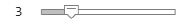128; [parameters,state] = initializexVecModelLayers(numFeatures,numFilters,numClasses)```
```parameters = struct with fields: conv1: [1×1 struct] batchnorm1: [1×1 struct] conv2: [1×1 struct] batchnorm2: [1×1 struct] conv3: [1×1 struct] batchnorm3: [1×1 struct] conv4: [1×1 struct] batchnorm4: [1×1 struct] conv5: [1×1 struct] batchnorm5: [1×1 struct] fc7: [1×1 struct] batchnorm7: [1×1 struct] fc8: [1×1 struct] batchnorm8: [1×1 struct] fc9: [1×1 struct] ```
```state = struct with fields: batchnorm1: [1×1 struct] batchnorm2: [1×1 struct] batchnorm3: [1×1 struct] batchnorm4: [1×1 struct] batchnorm5: [1×1 struct] batchnorm7: [1×1 struct] batchnorm8: [1×1 struct] ```

The table summarizes the architecture of the network described in  and implemented in this example. T is the total number of frames (feature vectors over time) in an audio signal. N is the number of classes (speakers) in the training set.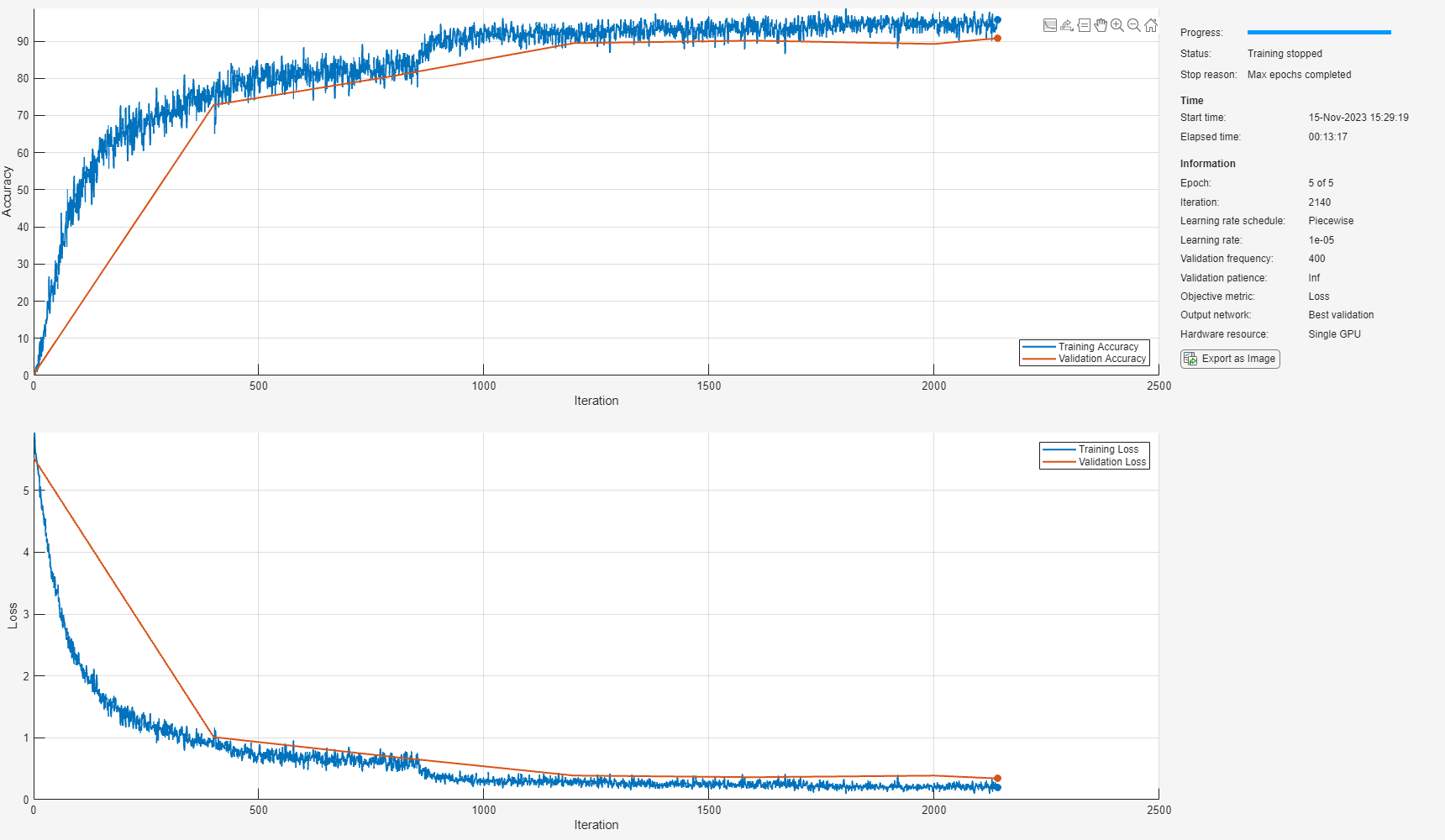### Train Model

Use `arrayDatastore` and `minibatchqueue` (Deep Learning Toolbox) to create a mini-batch queue for the training data.

```dsXTrain = arrayDatastore(XTrain,'OutputType','same'); dsYTrain = arrayDatastore(YTrain','OutputType','cell'); dsTrain = combine(dsXTrain,dsYTrain); miniBatchSize =128; numOutputs = 2; mbq = minibatchqueue(dsTrain,numOutputs, ... 'MiniBatchSize',miniBatchSize, ... 'MiniBatchFormat',{'SCB','CB'}, ... 'MiniBatchFcn',@preprocessMiniBatch);```

Set the number of training epochs, the initial learn rate, the learn rate drop period, the learn rate drop factor, and the validations per epoch.

```numEpochs =6; learnRate =0.001; gradDecay = 0.5; sqGradDecay = 0.999; trailingAvg = []; trailingAvgSq = []; LearnRateDropPeriod =2; LearnRateDropFactor =0.1; ValidationsPerEpoch =2; iterationsPerEpoch = floor(numel(XTrain)/miniBatchSize); iterationsPerValidation = round(iterationsPerEpoch/ValidationsPerEpoch);```

If you have access to a compute GPU, set `ExecutionEnvironment` to `gpu`. Otherwise, set `ExecutionEnvironment` to `cpu`.

`ExecutionEnvironment ='gpu';`

If performing validation while training, preprocess the validation set for faster in-the-loop performance.

```if ValidationsPerEpoch ~= 0 [XValidation,YValidation] = preprocessMiniBatch(XValidation,{YValidation}); XValidation = dlarray(XValidation,'SCB'); if strcmp(ExecutionEnvironment,'gpu') XValidation = gpuArray(XValidation); end end```

To display training progress, initialize the supporting object `progressPlotter`. The supporting object, `progressPlotter`, is placed in your current folder when you open this example.

Run the training loop.

```pp = progressPlotter(categories(classes)); iteration = 0; for epoch = 1:numEpochs % Shuffle mini-batch queue shuffle(mbq) while hasdata(mbq) % Update iteration counter iteration = iteration + 1; % Get mini-batch from mini-batch queue [dlX,Y] = next(mbq); % Place mini-batch on GPU if requested if strcmp(ExecutionEnvironment,'gpu') dlX = gpuArray(dlX); end % Evaluate the model gradients, state, and loss using dlfeval and the modelGradients function [gradients,state,loss,predictions] = dlfeval(@modelGradients,dlX,Y,parameters,state); % Update the network parameters using the Adam optimizer [parameters,trailingAvg,trailingAvgSq] = adamupdate(parameters,gradients, ... trailingAvg,trailingAvgSq,iteration,learnRate,gradDecay,sqGradDecay,eps('single')); % Update the training progress plot updateTrainingProgress(pp,'Epoch',epoch,'Iteration',iteration,'LearnRate',learnRate,'Predictions',predictions,'Targets',Y,'Loss',loss) % Update the validation plot if ~rem(iteration,iterationsPerValidation) % Pass validation data through x-vector model predictions = xvecModel(XValidation,parameters,state,'DoTraining',false); % Update plot updateValidation(pp,'Iteration',iteration,'Predictions',predictions,'Targets',YValidation) end end % Update learn rate if rem(epoch,LearnRateDropPeriod)==0 learnRate = learnRate*LearnRateDropFactor; end end```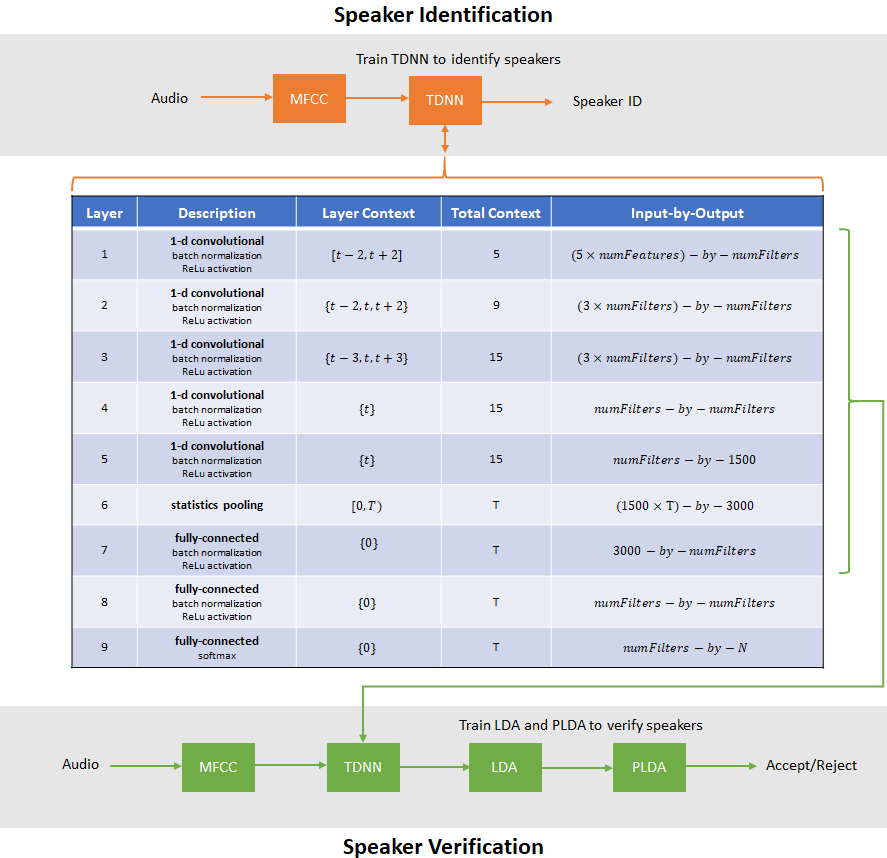### Evaluate TDNN Model

Evaluate the TDNN speaker recognition accuracy using the held-out test set. For each file in the test set:

1. Resample the audio to 16 kHz

2. Extract features using the `xVectorPreprocess` supporting function. Features are returned in cell arrays, where the number of elements in a cell array is equal to the number of individual speech segments.

3. To get the predicted speaker label, pass each segment through the model.

4. If more than one speech segment was present in the audio signal, average the predictions.

5. Use `onehotdecode` (Deep Learning Toolbox) to convert the prediction to a label.

Use `confusionchart` (Deep Learning Toolbox) to evaluate the system performance.

```predictedLabels = classes; predictedLabels(:) = []; for sample = 1:numel(adsTest.Files) [audioIn,xInfo] = read(adsTest); audioIn = resample(audioIn,desiredFs,fs); f = xVectorPreprocess(audioIn,afe,'Factors',factors,'MinimumDuration',0); predictions = zeros(numel(classes),numel(f)); for segment = 1:numel(f) dlX = dlarray(f{segment},'SCB'); predictions(:,segment) = extractdata(xvecModel(dlX,parameters,state,'DoTraining',false)); end predictedLabels(sample) = onehotdecode(mean(predictions,2),categories(classes),1); end trueLabels = adsTest.Labels; accuracy = mean(trueLabels==predictedLabels'); figure('Units','normalized','Position',[0.2 0.2 0.6 0.6]); confusionchart(trueLabels,predictedLabels', ... 'ColumnSummary','column-normalized', ... 'RowSummary','row-normalized', ... 'Title',sprintf('x-vector Speaker Recognition\nAccuracy = %0.2f%%',accuracy*100))```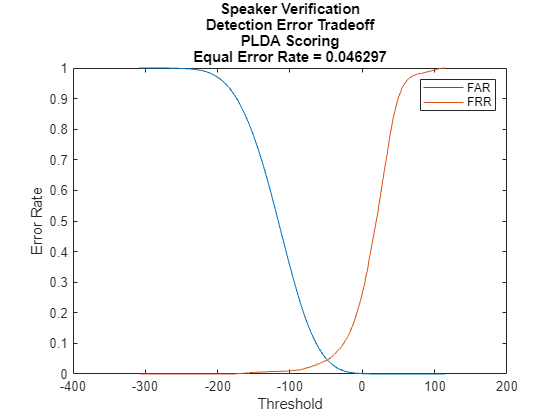### Train x-vector System Backend

In the x-vector system for speaker verification, the TDNN you just trained is used to output an embedding layer. The output from the embedding layer (layer 7 in this example, after batch normalization and before activation) are the 'x-vectors' in an x-vector system.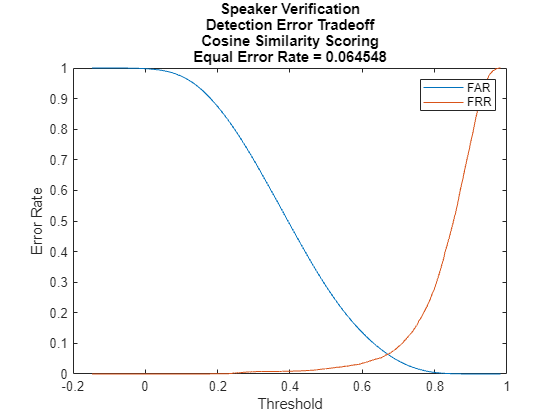The backend (or classifier) of an x-vector system is the same as the backend of an i-vector system. For details on the algorithms, see `ivectorSystem` and Speaker Verification Using i-Vectors.

Extract x-vectors from the train set. The supporting function, `xvecModel`, has the optional name-value pair `'OutputLayer'`. Set `'OutputLayer'` to `7` to return the output of the seventh layer. In , the output from either layer 7 or layer 8 are suggested as possible embedding layers.

```xvecs = zeros(numFilters,numel(YTrain)); for sample = 1:size(YTrain,2) dlX = dlarray(XTrain{sample},'SCB'); embedding = xvecModel(dlX,parameters,state,'DoTraining',false,'OutputLayer',7); xvecs(:,sample) = extractdata(embedding); end```

Create a linear discriminant analysis (LDA) projection matrix to reduce the dimensionality of the x-vectors to 32. LDA attempts to minimize the intra-class variance and maximize the variance between speakers.

```numEigenvectors =32; projMat = helperTrainProjectionMatrix(xvecs,YTrain,numEigenvectors);```

Apply the LDA projection matrix to the x-vectors.

`xvecs = projMat*xvecs;`

Train a G-PLDA model to perform scoring.

```numIterations =3; numDimensions =32; plda = helperTrainPLDA(xvecs,YTrain,numIterations,numDimensions);```

### Evaluate x-vector System

Speaker verification systems verify that a speaker is who they purport to be. Before a speaker can be verified, they must be enrolled in the system. Enrollment in the system means that the system has a template x-vector representation of the speaker.

#### Enroll Speakers

Extract x-vectors from the held-out data set, `adsEnroll`. Set the minimum duration of an audio segment to the equivalent of 15 features hops (the minimum number required to calculate x-vectors).

```minDur = (numel(afe.Window)+14*(numel(afe.Window)-afe.OverlapLength)+1)/desiredFs; xvecs = zeros(numEigenvectors,numel(adsEnroll.Files)); reset(adsEnroll) for sample = 1:numel(adsEnroll.Files) [audioIn,xInfo] = read(adsEnroll); audioIn = resample(audioIn,desiredFs,fs); f = xVectorPreprocess(audioIn,afe,'Factors',factors,'MinimumDuration',minDur); embeddings = zeros(numFilters,numel(f)); for segment = 1:numel(f) dlX = dlarray(f{segment},'SCB'); embeddings(:,segment) = extractdata(xvecModel(dlX,parameters,state,'DoTraining',false,'OutputLayer',7)); end xvecs(:,sample) = mean(projMat*embeddings,2); end```

Create template x-vectors for each speaker by averaging the x-vectors of individual speakers across enrollment files.

```labels = adsEnroll.Labels; uniqueLabels = unique(labels); atable = cell2table(cell(0,2),'VariableNames',{'xvector','NumSamples'}); for ii = 1:numel(uniqueLabels) idx = uniqueLabels(ii)==labels; wLocalMean = mean(xvecs(:,idx),2); localTable = table({wLocalMean},(sum(idx)), ... 'VariableNames',{'xvector','NumSamples'}, ... 'RowNames',string(uniqueLabels(ii))); atable = [atable;localTable]; %#ok<AGROW> end enrolledLabels = atable```
```enrolledLabels=2×2 table xvector NumSamples _____________ __________ F05 {32×1 double} 3 M05 {32×1 double} 3 ```

Speaker verification systems require you to set a threshold that balances the probability of a false acceptance (FA) and the probability of a false rejection (FR), according to the requirements of your application. To determine the threshold that meets your FA/FR requirements, evaluate the detection error tradeoff of the system.

```xvecs = zeros(numEigenvectors,numel(adsDET.Files)); reset(adsDET) for sample = 1:numel(adsDET.Files) [audioIn,xInfo] = read(adsDET); audioIn = resample(audioIn,desiredFs,fs); f = xVectorPreprocess(audioIn,afe,'Factors',factors,'MinimumDuration',minDur); embeddings = zeros(numFilters,numel(f)); for segment = 1:numel(f) dlX = dlarray(f{segment},'SCB'); embeddings(:,segment) = extractdata(xvecModel(dlX,parameters,state,'DoTraining',false,'OutputLayer',7)); end xvecs(:,sample) = mean(projMat*embeddings,2); end labels = adsDET.Labels; [detTable,scoreRange] = helperDetectionErrorTradeoff(xvecs,labels,enrolledLabels,plda);```

Plot the results of the detection error tradeoff evaluation for both PLDA scoring and cosine similarity scoring (CSS).

```plot(detTable.PLDA.Threshold,detTable.PLDA.FAR, ... detTable.PLDA.Threshold,detTable.PLDA.FRR) eer = helperEqualErrorRate(detTable.PLDA); title(sprintf('Speaker Verification\nDetection Error Tradeoff\nPLDA Scoring\nEqual Error Rate = %0.2f',eer)); xlabel('Threshold') ylabel('Error Rate') legend({'FAR','FRR'})``````plot(detTable.CSS.Threshold,detTable.CSS.FAR, ... detTable.CSS.Threshold,detTable.CSS.FRR) eer = helperEqualErrorRate(detTable.CSS); title(sprintf('Speaker Verification\nDetection Error Tradeoff\nCosine Similarity Scoring\nEqual Error Rate = %0.2f',eer)); xlabel('Threshold') ylabel('Error Rate') legend({'FAR','FRR'})```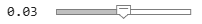### References

 Snyder, David, et al. “x-vectors: Robust DNN Embeddings for Speaker Recognition.” 2018 IEEE International Conference on Acoustics, Speech and Signal Processing (ICASSP), IEEE, 2018, pp. 5329–33. DOI.org (Crossref), doi:10.1109/ICASSP.2018.8461375.

 Signal Processing and Speech Communication Laboratory. Accessed December 12, 2019. https://www.spsc.tugraz.at/databases-and-tools/ptdb-tug-pitch-tracking-database-from-graz-university-of-technology.html.

### Supporting Functions

#### Initialize Parameters of TDNN Layers

```function [parameters,state] = initializexVecModelLayers(numFeatures,numFilters,numClasses) % This function is only for use in this example. It may be changed or % removed in a future release. % Initialize Layer 1 (1-D Convolutional) filterSize1 = 5; numChannels1 = numFeatures; numFilters1 = numFilters; numIn1 = filterSize1*numFilters1; numOut1 = filterSize1*numFilters1; parameters.conv1.Weights = initializeGlorot([filterSize1,numChannels1,numFilters1],numOut1,numIn1); parameters.conv1.Bias = dlarray(zeros([numFilters1,1],'single')); parameters.batchnorm1.Offset = dlarray(zeros([numFilters1,1],'single')); parameters.batchnorm1.Scale = dlarray(ones([numFilters1,1],'single')); state.batchnorm1.TrainedMean = zeros(numFilters1,1,'single'); state.batchnorm1.TrainedVariance = ones(numFilters1,1,'single'); % Initialize Layer 2 (1-D Convolutional) filterSize2 = 3; numChannels2 = numFilters1; numFilters2 = numFilters; numIn2 = filterSize2*numFilters2; numOut2 = filterSize2*numFilters2; parameters.conv2.Weights = initializeGlorot([filterSize2,numChannels2,numFilters2],numOut2,numIn2); parameters.conv2.Bias = dlarray(zeros([numFilters2,1],'single')); parameters.batchnorm2.Offset = dlarray(zeros([numFilters2,1],'single')); parameters.batchnorm2.Scale = dlarray(ones([numFilters2,1],'single')); state.batchnorm2.TrainedMean = zeros(numFilters2,1,'single'); state.batchnorm2.TrainedVariance = ones(numFilters2,1,'single'); % Initialize Layer 3 (1-D Convolutional) filterSize3 = 3; numChannels3 = numFilters2; numFilters3 = numFilters; numIn3 = filterSize3*numFilters3; numOut3 = filterSize3*numFilters3; parameters.conv3.Weights = initializeGlorot([filterSize3,numChannels3,numFilters3],numOut3,numIn3); parameters.conv3.Bias = dlarray(zeros([numFilters3,1],'single')); parameters.batchnorm3.Offset = dlarray(zeros([numFilters3,1],'single')); parameters.batchnorm3.Scale = dlarray(ones([numFilters3,1],'single')); state.batchnorm3.TrainedMean = zeros(numFilters3,1,'single'); state.batchnorm3.TrainedVariance = ones(numFilters3,1,'single'); % Initialize Layer 4 (1-D Convolutional) filterSize4 = 1; numChannels4 = numFilters3; numFilters4 = numFilters; numIn4 = filterSize4*numFilters4; numOut4 = filterSize4*numFilters4; parameters.conv4.Weights = initializeGlorot([filterSize4,numChannels4,numFilters4],numOut4,numIn4); parameters.conv4.Bias = dlarray(zeros([numFilters4,1],'single')); parameters.batchnorm4.Offset = dlarray(zeros([numFilters4,1],'single')); parameters.batchnorm4.Scale = dlarray(ones([numFilters4,1],'single')); state.batchnorm4.TrainedMean = zeros(numFilters4,1,'single'); state.batchnorm4.TrainedVariance = ones(numFilters4,1,'single'); % Initialize Layer 5 (1-D Convolutional) filterSize5 = 1; numChannels5 = numFilters4; numFilters5 = 1500; numOut5 = filterSize5*numFilters5; numIn5 = filterSize5*numFilters5; parameters.conv5.Weights = initializeGlorot([filterSize5,numChannels5,numFilters5],numOut5,numIn5); parameters.conv5.Bias = dlarray(zeros([numFilters5,1],'single')); parameters.batchnorm5.Offset = dlarray(zeros([numFilters5,1],'single')); parameters.batchnorm5.Scale = dlarray(ones([numFilters5,1],'single')); state.batchnorm5.TrainedMean = zeros(numFilters5,1,'single'); state.batchnorm5.TrainedVariance = ones(numFilters5,1,'single'); % Initialize Layer 6 (Statistical Pooling) numIn6 = numOut5; numOut6 = 2*numIn6; % Initialize Layer 7 (Fully Connected) numIn7 = numOut6; numOut7 = numFilters; parameters.fc7.Weights = initializeGlorot([numFilters,numIn7],numOut7,numIn7); parameters.fc7.Bias = dlarray(zeros([numOut7,1],'single')); parameters.batchnorm7.Offset = dlarray(zeros([numOut7,1],'single')); parameters.batchnorm7.Scale = dlarray(ones([numOut7,1],'single')); state.batchnorm7.TrainedMean = zeros(numOut7,1,'single'); state.batchnorm7.TrainedVariance = ones(numOut7,1,'single'); % Initialize Layer 8 (Fully Connected) numIn8 = numOut7; numOut8 = numFilters; parameters.fc8.Weights = initializeGlorot([numOut8,numIn8],numOut8,numIn8); parameters.fc8.Bias = dlarray(zeros([numOut8,1],'single')); parameters.batchnorm8.Offset = dlarray(zeros([numOut8,1],'single')); parameters.batchnorm8.Scale = dlarray(ones([numOut8,1],'single')); state.batchnorm8.TrainedMean = zeros(numOut8,1,'single'); state.batchnorm8.TrainedVariance = ones(numOut8,1,'single'); % Initialize Layer 9 (Fully Connected) numIn9 = numOut8; numOut9 = numClasses; parameters.fc9.Weights = initializeGlorot([numOut9,numIn9],numOut9,numIn9); parameters.fc9.Bias = dlarray(zeros([numOut9,1],'single')); end```

#### Initialize Weights Using Glorot Initialization

```function weights = initializeGlorot(sz,numOut,numIn) % This function is only for use in this example. It may be changed or % removed in a future release. Z = 2*rand(sz,'single') - 1; bound = sqrt(6 / (numIn + numOut)); weights = bound*Z; weights = dlarray(weights); end```

#### Calculate Model Gradients and Updated State

```function [gradients,state,loss,Y] = modelGradients(X,target,parameters,state) % This function is only for use in this example. It may be changed or % removed in a future release. [Y,state] = xvecModel(X,parameters,state,'DoTraining',true); loss = crossentropy(Y,target); gradients = dlgradient(loss,parameters); end```

#### Preprocess Mini-Batch

```function [sequences,labels] = preprocessMiniBatch(sequences,labels) % This function is only for use in this example. It may be changed or % removed in a future release. lengths = cellfun(@(x)size(x,1),sequences); minLength = min(lengths); sequences = cellfun(@(x)randomTruncate(x,1,minLength),sequences,'UniformOutput',false); sequences = cat(3,sequences{:}); labels = cat(2,labels{:}); labels = onehotencode(labels,1); labels(isnan(labels)) = 0; end```

#### Randomly Truncate Audio Signals to Specified Length

```function y = randomTruncate(x,dim,minLength) % This function is only for use in this example. It may be changed or % removed in a future release. N = size(x,dim); if N > minLength start = randperm(N-minLength,1); if dim==1 y = x(start:start+minLength-1,:); elseif dim ==2 y = x(:,start:start+minLength-1); end else y = x; end end```

#### Feature Extraction and Normalization - Datastore

```function [features,labels] = xVectorPreprocessBatch(ads,afe,nvargs) % This function is only for use in this example. It may be changed or % removed in a future release. arguments ads afe nvargs.Factors = [] nvargs.Segment = true; end if ~isempty(ver('parallel')) pool = gcp; numpar = numpartitions(ads,pool); else numpar = 1; end labels = []; features = []; parfor ii = 1:numpar adsPart = partition(ads,numpar,ii); numFiles = numel(adsPart.UnderlyingDatastores{1}.Files); localFeatures = cell(numFiles,1); localLabels = []; for jj = 1:numFiles [audioIn,xInfo] = read(adsPart); label = xInfo.Label; [f,ns] = xVectorPreprocess(audioIn,afe,'Factors',nvargs.Factors,'Segment',nvargs.Segment); %#ok<PFBNS> localFeatures{jj} = f; localLabels = [localLabels,repelem(label,ns)]; end features = [features;localFeatures]; labels = [labels,localLabels]; end features = cat(1,features{:}); labels = removecats(labels); end```

#### Feature Extraction and Normalization

```function [features,numSegments] = xVectorPreprocess(audioData,afe,nvargs) % This function is only for use in this example. It may be changed or % removed in a future release. arguments audioData afe nvargs.Factors = [] nvargs.Segment = true; nvargs.MinimumDuration = 1; end % Scale audioData = audioData/max(abs(audioData(:))); % Protect against NaNs audioData(isnan(audioData)) = 0; % Determine regions of speech mergeDur = 0.5; % seconds idx = detectSpeech(audioData,afe.SampleRate,'MergeDistance',afe.SampleRate*mergeDur); % If a region is less than MinimumDuration seconds, drop it. if nvargs.Segment idxToRemove = (idx(:,2)-idx(:,1))<afe.SampleRate*nvargs.MinimumDuration; idx(idxToRemove,:) = []; end % Extract features numSegments = size(idx,1); features = cell(numSegments,1); for ii = 1:numSegments features{ii} = single(extract(afe,audioData(idx(ii,1):idx(ii,2)))); end % Standardize features if ~isempty(nvargs.Factors) features = cellfun(@(x)(x-nvargs.Factors.Mean)./nvargs.Factors.STD,features,'UniformOutput',false); end % Cepstral mean subtraction (for channel noise) if ~isempty(nvargs.Factors) fileMean = mean(cat(1,features{:}),'all'); features = cellfun(@(x)x - fileMean,features,'UniformOutput',false); end if ~nvargs.Segment features = cat(1,features{:}); end end```

## SupportGet trial now